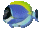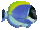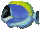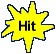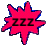Solve Equation Types:   ay+b=cy+d  ay-b=cy+d       Math Fun Game Tips:

- To solve 5y+7 = 2y+19, the first step is to collect the y terms together on the left side.
To remove 2y from the right side 2y+19, you subtract 2y from each side.
The first step can be written as 5y-2y+7 = 2y-2y+19 which yields the result 3y+7 = 19.
The next step can be written as 3y+7-7 = 19-7 which simplifies to 3y = 12 .
The final step uses the method 3y/3 = 12/3 to isolate the y on one side, giving the answer y = 4.

- For equation 5y+7 = 2y+19, the answer y=4 is the value of the variable y that satisfies the equation.
To satisfy an equation means to make its left side value equal its right side value.
When you check the answer y=4, the left side value of 5y+7 evaluates as 5(4)+7 which is 27.
When you check the right side value of 2y+19, it evaluates as 2(4)+19 which is 27.

- The answer y=4 that satisfies the equation 5y+7 = 2y+19 is the solution or root of the equation.

- Similarly, a complete solution for solving the equation 7w-8 = 4w+22 to find the value of w is ...
7w-8 = 4w+22
7w-4w-8 = 4w-4w+22
3w-8 = 22
3w-8+8 = 22+8
3w = 30
3w/3 = 30/3
w = 10

- Your Game Score is reduced by the number of fish hits.

- To slow the game speed repeat tap/click on the word Slider.
- To increase the game speed repeat tap/click on the word Math.
- Speed can also be adjusted with a keyboard's - and + keys.

- Refresh/Reload the web page to restart the game.